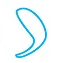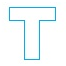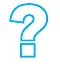# Indian Talent Olympiad - NMITO PDF Sample Papers for Class 7

Resources:

Class 7 sample paper & practice questions for National Mathematics Indian Talent Olympiad (NMITO) level 1 are given below. Syllabus for level 1 is also mentioned for these exams. You can refer these sample paper & quiz for preparing for the exam.Q.1 Q.2 Q.3 Q.4 Q.5 Q.6 Q.7 Q.8 Q.9 Q.10
 Q.1 Which of the following has a line of symmetry? a)b)c)d)Q.2 Three candidates contested an election and received 1136, 7636 and 11628 votes respectively. What percentage of the total votes did the winning candidate got? a) 58% b) 56% c) 57% d) 55%
 Q.3 What are two positive numbers differ by 5 and square of their sum is 169? a) 5, 10 b) 3, 8 c) 6, 11 d) 4, 9
 Q.4 Name the solid that has two opposite identical faces and other faces as parallelogram. a) Pyramid b) Prism c) Sphere d) Cone
 Q.5 Find the measure of an angle which is four times its supplement. a) 154° b) 64° c) 144° d) 124°
 Q.6 Which of the following fractions is greater than 3/4 and less than 5/6? a) 1/2 b) 4/5 c) 2/3 d) 9/10
 Q.7 For 71 which symbol is used in Roman system? a) LXXI b) XLI c) LXXXI d) LI
 Q.8 What is the total number of edges a cylinder has? a) 0 b) 1 c) 3 d) 2
 Q.9 Find the mirror image of the following:a) 1 b) 2 c) 3 d) 4
 Q.10 Fill in the blanks. If a, b and c are integers, then according to associative law of multiplication (a x b) x c must be equal to __. a) a x ( b + c ) b) a x b + a x c c) ( a - b ) x c d) a x (b x c)Sample PDF of Indian Talent Olympiad - National Mathematics Indian Talent Olympiad (NMITO) PDF Sample Papers for Class 7: